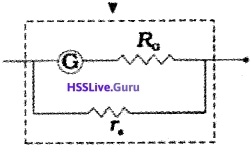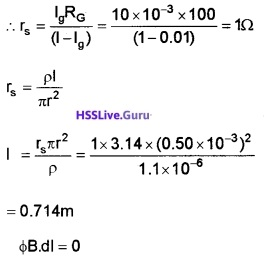# Plus Two Physics Chapter Wise Questions and Answers Chapter 4 Moving Charges and Magnetism

Students can Download Chapter 4 Moving Charges and Magnetism Questions and Answers, Plus Two Physics Chapter Wise Questions and Answers helps you to revise the complete Kerala State Syllabus and score more marks in your examinations.

## Kerala Plus Two Physics Chapter Wise Questions and Answers Chapter 4 Moving Charges and Magnetism

### Plus Two Physics Moving Charges and Magnetism NCERT Text Book Questions and Answers

Question 1.
A Circular coil of wire consisting of 100 turns, each of radius 8.0cm carries a current of 0.40A. What is the magnitude of the magnetic field B at the centre of the coil?
Given n = 100, r = 8.0cm = 8 × 10-2
I = 0.4A, B = ?
At the centre of circular coil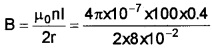= π × 104T = 3.1 × 10-4T.Question 2.
A uniform field equal to 1.5T exists in a cylindrical region of radius 10.0cm, its direction parallel to the axis along east to west. A wire carrying current of 7.0A in the north to south direction passes through this region. What is the magnitude and direction of the force on the wire if

1. The wire intersects the axis?
2. The wire is turned from N-S to north-east-north-west direction.
3. The wire in the N-S direction is lowered from the axis by a distance of 4.0cm?

1. F = $$\mathrm{Bl} \ell$$ = 1.5 × 7 × $$\frac{20}{100}$$ or F = 2.1 N acting vertically downwards.

2. Force will again be 2.1N.

3. F = $$\frac{1.5 \times 7 \times 16}{100}$$ = 1.68N.

Question 3.
Two long and parallel straight wires A and B carrying currents of 8.0A and 5.0A in the same direction are separated by a distance of 4.0cm. Estimate the force on a 10cm section of wire A.
Given I1 = 8.0A, l2 = 5.0A, r = 4.0cm = 0.04m
l = 10cm = 0.10m
Since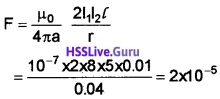(direction is given by Fleming left-hand rule).

### Plus Two Physics Moving Charges and Magnetism One Mark Questions and Answers

Question 1.
A straight wire of length 0.5 metre and carrying a current of 1.2 ampere is placed in uniform magnetic field of induction 2 tesla. The magnetic field is perpendicular to the length of the wire. Find the force on the wire is
F = i/B = 1.2 × 0.5 × 2 = 1.2 NQuestion 2.
To convert a galvanometer into a ammeter, one needs to connect a.
(a) low resistance in parallel
(b) high resistance in parallel
(c) low resistance in series
(d) high resistance in series
(a) low resistance in parallel

Question 3.
A coil carrying electric current is placed in uniform magnetic field.
(a) torque is formed
(b) e.m.f is induced
(c) both a and b are correct
(d) none of these
(a) torque is formedQuestion 4.
Direction of motion of unit the positive test charge gives direction electric field. Direction of motion of…….gives direction of magnetic field.
Unit north pole.

Question 5.
A magnetic system with zero dipole moment
(a) Solenoid
(b) current carrying coil
(c) current loop
(d) toroid
(d) Toroid.

Question 6.
Find odd one regarding polarity solenoid, torroid, current carrying loop, bar magnet.
Torroid (In this case North and South pole are absent).Question 7.
The nature of path when a charged particle is projected 30° to the direction of magnetic field. (Helix, cycloid, straight line, parabola).
Helix

Question 8.
What is solenoid?
An insulated copper wire wound in the form of cylinder is called solenoid.

Question 9.
Write mathematical expression for ampere’s theorem.
∫B.dl = µ0I

### Plus Two Physics Moving Charges and Magnetism Two Mark Questions and Answers

Question 1.
The figure shows a long straight conductor carrying a current I. A magnetic field is produced around the conductor.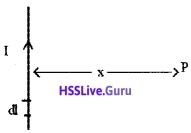What is the magnitude of the magnetic induction at a point ‘P’ which is at a distant ‘x’ from the conductor?
What is the shape of the magnetic line of force?

1. B = $$\frac{\mu_{0} I}{2 \pi x}$$. Magnetic field is directed in to the plane of paper.

2. Circular.Question 2.
A particle of mass 6.65 × 10-27 kg having positive charge equal to two times of electron, moves with a speed of 6 × 105 m/s in a direction perpendicular to that of a given magnetic field of flux density 0.4 weber/m2. Find the acceleration of the particle.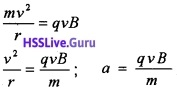q = 2e, v = 6 × 105 m/s
B = 0.4, m = 6.65 × 10-27 kg
∴ acceleration,a = 1.15 × 1013 m/s2.

Question 3.
Classify in to true or false.

1. The magnetic field in the middle of current carrying solenoid depends up on cross sectional area.
2. The magnetic field depends up on current.
3. The magnetic field depends up on the material of the core.
4. The magnetic field depends up on total numbers of turns per unit length.

1. False
2. True
3. True
4. TrueQuestion 4.
A short straight conductor carries current I

1. Write the expression for magnetic field due to this conductor.
2. Represent graphically the variation of magnetic field with distance from the wire.

1. dB = $$\frac{\mu_{0}}{4 \pi} \frac{|d| \sin \theta}{r^{2}}$$

2.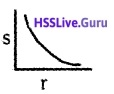### Plus Two Physics Moving Charges and Magnetism Three Mark Questions and Answers

Question 1.
The magnetic field along the axis of a circular coil is found to be B = $$\frac{\mu_{\mathrm{o}} \mathrm{Ia}^{2}}{2\left(\mathrm{r}^{2}+\mathrm{a}^{2}\right)^{3 / 2}}$$
1. What is the magnetic field along the axis if r>>a
2.

• Compare the above magnetic field with the electric field along the axis of an electric dipole
• What is the equation of magnetic dipole moment?

1. B = $$\frac{\mu_{0} / a^{2}}{2 r^{3}}$$

2.

• Electric field due to electric dipole, E = $$\frac{1}{4 \pi \varepsilon_{0}} \frac{P}{r^{3}}$$ magnetic field due to magnetic dipole B = $$\frac{\mu_{0}}{4 \pi} \frac{m}{x^{3}}$$.
• Magnetic moment m = IAQuestion 2.
The circuit diagram for verifying Ohm’s Law is given below, A student unknowingly connects a galvanometer in the place of the ammeter.1. What will happen to the galvanometer?
2. What modification has to be made in the galvanometer if he still wants to use the galvanometer in place of the ammeter?
3. Assuming voltmeter to be an ideal one, what will happen if the student interchanges the position of the voltmeter and ammeter?

1. Galvanometer will be damaged.
2. use a shunt resistance.
3. No current flows, because an ideal voltmeter has infinite resistance.Question 3.
Consider a galvanometer with a full scale deflection of 1 m A and resistance 100Ω.

1. How is the device connected in the circuit?
2. How can it be converted to an ammeter with full scale deflection 1 ampere?

1. Connected in series.

2.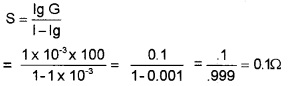by connecting a shunt resistance. 0.1Ω in parallel with galvanometer, we can convert galvanometer in to ammeter.

Question 4.
Two infinitely long straight parallel wires carry currents I each as shown in fig.

1. Which law helps to find direction of magnetic field around a current carrying conductor?
2. What is the magnitude, direction of the magnetic fields at A, and C?1. Right hand grip rule.

2. The magnitude, direction of the magnetic fields at A, and C:

• A – outward to the plane of paper
• C-Inward to the plane of paper.Question 5.
Analyze the figure and answer the following questions.

1. What is the nature of force between these conductors is……….
2. What is the field due to I1 at second conductor?
3. What is the force experienced per unit length of IInd conductor?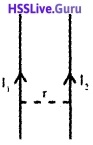1. Attractive
2. B= $$\frac{\mu_{0} d_{1}}{2 \pi r}$$
3. f = $$\frac{\mu_{0} l_{1} l_{2}}{2 \pi r}$$Question 6.
“I am very light and present in every matter. When I move along the equator from east to west, I am pushed up. When I am stationary, no force”.

1. Name the force and write its expression.
2. Who am I?
3. What would happen if moved at the poles?

1. Lorentz force in earth’s magnetic field F =qvB
2. Electron
3. The earth’s magnetic field at poles is perpendicular to the earth surface. When an electron move, it is pushed to one side, parallel to earth’s surface.

### Plus Two Physics Moving Charges and Magnetism Four Mark Questions and Answers

Question 1.
The internal connections of a moving coil galvanometer is given in the fig (i) and fig (ii)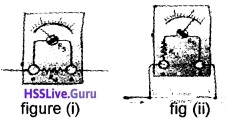1. What is the use of moving coil galvanometer?
2. Which figure(i) or figure(ii) is used to measure Voltage?
3. Write an expression for resistance required to convert the moving coil galvanometer in to voltmeter.
4. If a very small resistance (eg, copper wire) is used to convert moving coil galvanometer in to voltmeter, will it work properly?

1. Moving coil galvanometer is used to detect the presence of current
2. The instrument shown in figure (2) is used to measure voltage.
3. Resistance is connected in series with galvanometer. R = $$\frac{\mathrm{V}}{\mathrm{I}_{\mathrm{g}}}$$ – G
4. No, A high resistance is required to convert galvanometer in to voltmeter.Question 2.
When a charged particle enter normal to a uniform magnetic field, it take a circular path.

1. Name the particle accelerator using this principle.
2. Explain the working of that particle accelerator with relevant theory.
3. The neutrons can’t be accelerated using this partide accelerator. Why?

1. Cyclotron.

2. Cyclotron:
a. Uses:
It is a device used to accelerate particles to high energy.

b. Principles:
Cyclotron is based on two facts

• An electric field can accelerate a charged particle.
• A perpendicular magnetic field gives the ion a circular path.

c. Working: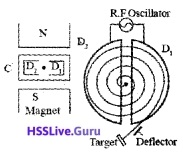At certain instant, let D1 be positive and D2 be negative. Ion (+ve) will be accelerated towards D2 and describes a semicircular path (inside it). When the particle reaches the gap, D1 becomes negative and D2 becomes positive.

So ion is accelerated towards D1 and undergoes a circular motion with larger radius. This process repeats again and again. Thus ion comes near the edge of the dee with high K.E. This ion can be directed towards the target by a deflecting plate.

3. Charge of neutron is zero. Only charged particle can be accelerated using particle accelerated.Question 3.
A current flows through a circular loop of radius r is shown in the figure.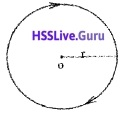1. What is the direction of magnetic field at ‘o’? (1)

2. Derive an equation for magnetic field at ‘o’ due to the circular loop carrying current i? (2)

3. If the loop splits into two equal halves as shown in figure. (1)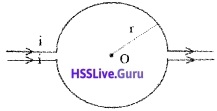What will be the magnetic field at the center ‘o’?
1. B = $$\frac{\mu_{0} I}{2 r}$$ in t0 the plane of paper

2. Magnetic field on the axis of a circular current loop:
Consider a circular loop of radius ‘a’ and carrying current T. Let P be a point on the axis of the coil, at distance x from A and r from ‘O’. Consider a small length dl at A.
The magnetic field at ‘p’ due to this small element dl,
dB =$$\frac{\mu_{0} \mathrm{Idl} \sin 90}{4 \pi \mathrm{x}^{2}}$$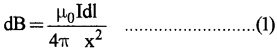[since sin 90° -1]
The dB can be resolved into dB cosΦ (along Py) and dB sinΦ (along Px). Similarly consider a small element at B, which produces a magnetic field ‘dB’ at P. If we resolve this magnetic field we get.
dB sinΦ (along px) and dB cosΦ (along py1)
dB cosΦ components cancel each other, because they are in opposite direction. So only dB sinΦ components are found at P, so total filed at P is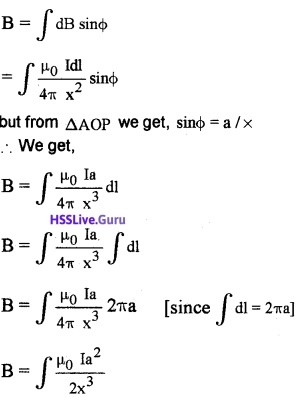from ∆AOP we get x = (r2 + a2 )1/2Let there be N turns in the loop then,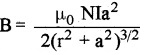Point at the centre of the loop:
When the point is at the centre of the loop, (r = 0) Then,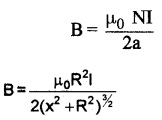3. ZeroQuestion 4.
A boy connects a galvanometer directly to a cell of emf 1.5v to measure a current through a load 1Ω.

1. Which instrument can be used to measure the current in such a circuit?
2. What changes should be made in the galvanometer to measure such a high current? Explain using a circuit diagram.
3. The boy connected the galvanometer into a high current measuring device and he connected the device parallel to the load. What will be the observation. Justify.

1. Ammeter

2.A galvanometer can be converted into an ammeter by a low resistance (shunt) connected parallel to it.
Theory:
Let G be the resistance of the galvanometer, giving full deflection fora current Ig. To convert it into an ammeter, a suitable shunt resistance ‘S’ is connected in parallel. In this arrangement, Ig current flows through Galvanometer and remaining (I-Ig) current flows through shunt resistance.
Since G and S are parallel
P.d Across G = p.d across S
Ig × G =(I-Ig)S
S = $$\frac{\lg \mathrm{G}}{\left(1-\mathrm{l}_{\mathrm{g}}\right)}$$
Connecting this shunt resistance across galvanometer we can convert a galvanometer into ammeter.

3. Ammeter is a low resistance device. Hence it draws high current. This high current will damage it.

Question 5.
An electric charge will experience a force in uniform electric field. Similarly a moving charge experience a magnetic force (Lorentz) in magnetic field. The SI unit of magnetic field intensity is defined in terms of Lorentz Force.

1. Write the expression for magnetic Lorentz force.
2. Mention any two difference between electric field arid magnetic field.
3. Give an account of work done by Magnetic Lorentz force on moving charge and corresponding change in K.E.

1.2. Electric field is due to a charge, either in motion or at rest. Magnetic field is due to the motion of charge. Direction of electric force is colinear to electric field. Direction of magnetic force is perpendicularto magnetic field.

3. If velocity (displacement) is perpendicular to Lorentz force the work done will be zero and hence there will no change in K.E.Question 6.
“Moving coil Galvanorrieter is a device used for detecting very feeble current”.

1. What is the working principle of a moving coil galvanometer?
2. Describe the construction and working of a moving coil galvanometer.
3. When a high current is passed through a moving coil galvanometer, it will get destroyed. How?

1. Principle:
A conductor carrying current when placed in a magnetic field experiences a force, (given by Fleming’s left hand rule), τ = NIAB.

2.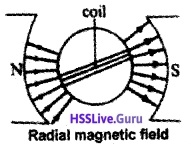A moving coil galvanometer consists of rectangular coil of wire having area ‘A’ and number of turns ‘n’ which is wound on metallic frame and is placed between two magnets. The magnets are concave in shape, which produces radial field.
Working :
Let T be the current flowing the coil, Then the torque acting on the coil. τ = NIAB, Where A is the area of coil and B is the magnetic field.

This torque produces a rotation on coil, thus fiber is twisted and angle (Φ). Due to this twisting a restoring torque (τ = KΦ) is produced in spring. Under equilibrium, we can write
Torque on the coil = restoring torque on the spring
NIAB = KΦ
Φ $$=\left(\frac{\mathrm{BAN}}{\mathrm{K}}\right)$$ I
The quantity inside the bracket is constant for a galvanometer.
Φ ∝ I
The above equation shows that the deflection depends on current passing through galvanometer.

3. High current will produce large amount of heat. This heat will destroy coils in the galvanometer.Question 7.
When a current carrying conductor is placed in a magnetic field it experiences a force.
1. Arrive at the expression forthe force experienced by the conductor. (2)
2. A conductor carrying current I direct out of the plane of the paper is lying in the magnetic field as in Fig. Draw the direction of force experienced by the conductor. (1)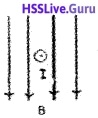3. If the conductor is lying parallel to the field what will be the force? (2)
1. Consider a rod of uniform cross section ‘A’ and length ‘l’ Let ‘n’ be the number of electrons per unit volume (number density). ‘vd’ be the drift velocityof electrons for steady current ‘I’.
Total number of electrons in the entire volume of rod =nAl
Charge of total electrons = nA l .e
‘e’ is the charge of a single electron.
The Lorentz force on electrons,2.3. ZeroQuestion 8.
A long straight conductor carrying current is placed near a current carrying circular loop as in the figure.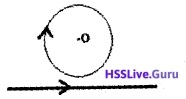1. If B1 is the field of the ring and B2 the field due to straight conductor what will be the direction of B1 and B2 at O. (1)

2. The current through the loop and the conductor are 2A and the conductor is at as distance 20cm from the centre of loop. What should be the diameter of the loop so that the net field at O is zero. (3)
1. B1 into the plane and B2 out of the plane.

2. B1 is the field of the ring and B2 the field of due to straight conductor.
B = B1 – B2 = 0
B1 = B2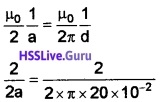2a = 40π × 10-2m = 1,256m
Diameter d = 1.256 m.Question 9.
A charged particle is travelling in the figure.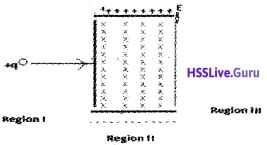1. Name the force experienced by the charge in the region II. (1)
2. Give the expression for the net force experienced by the charge in the region II. (1)
3. If the charge reaches the region III without any change in its initial direction of motion find the velocity of the charged particle in terms of E and B. (2)

1. Lorentz Force

2. F = q (E + v × B)

3. Electric and magnetic fields are perpendicular to each other and also perpendicular to the velocity of the particle. If the total force on the charge is zero and the charge will move in the fields undeflected. This happens when
qE = qvB or v = $$\frac{E}{B}$$.Question 10.
The medical diagnostic technique called magnetic resonance imaging (MRI) requires that patient lie in a strong magnetic field. It consists of two large solenoids, placed above and below.

1. What is solenoid?
2. Which law help you to find magnetic field due to solenoid? State the law.
3. Obtain an expression for magnetic field due to solenoid using the above law.
4. If the diameter of one of the MRI coil is increased without changing the current, does the magnetic field that it produce at its centre increases, decreases or stay the same? Justify.

1. An insulated conducting wire wound in the form of cylinder is called solenoid.

2. Ampere’s circuital law:
Ampere’s circuital theorem states that the line integral of the magnetic field around any closed path in free space is equal to µ0 times the net current passing through the surface.

3.Consider a solenoid having radius Y. Let ‘n’ be the number of turns per unit length and I be the current flowing through it.

In order to find the magnetic field (inside the solenoid ) consider an Amperian loop PQRS. Let ‘l‘ be the length and ‘b’ the breadthApplying Amperes law, we can write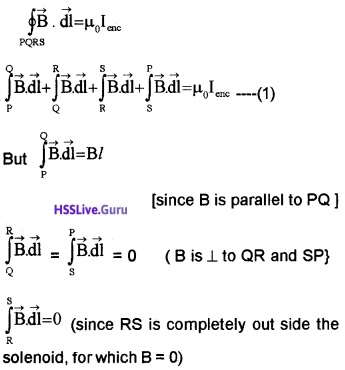Substituting the above values in eq (1), we get
Bl = µ0 lenc (2)
But lenc = n l I
where ’nl ’ is the total number of turns that carries current I (inside the loop PQRS)
∴ eq (2) can be written as
Bl = µ0nIl
B = µ0 nI
If core of solenoid is filled with a medium of relative permittivity µr, then
B = µ0µrnl

4. No change. Magnetic field is independent of radius.

### Plus Two Physics Moving Charges and Magnetism Five Mark Questions and Answers

Question 1.
You are supplied with a galvanometer, resistor, and some connecting wires.

1. Using a circuit diagram, show how will you convert the given galvanometer into an ammeter.
2. Find the expression for the shunt resistance in the circuit.
3. A galvanometer is to be converted into an ammeter of range 0 – 1 A. Galvanometer has resistance 100Ω and the current for full scale deflection is 10mA. Find the length of the nichrome wire to be used as shunt.

Given, Resistivity ρ= 1.1 x 10-6Ωm
Diameter of the wire = 1mm
1.2. Let Ig be the current through the galvanometer of resistance RG and the shunt resistance be rs.
Let I be the current to be measured by the converted ammeter.
We can write,
IgRG = (I – Ig)rs
∴ rs = $$\frac{I_{g} R_{G}}{\left(1-I_{g}\right)}$$

3. Given I = 1A
Ig = 10mA
RG = 100Ω
Diameter = 1mmQuestion 2.
Consider a conductor carrying current ‘I’, P is a point at a distance away from the conductor.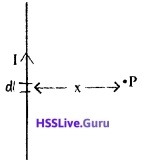1. What is the direction of magnetic field at P? (1)
2. What are the factors affecting magnetic field at P due the element dl carrying current i? (1)
3. Derive an expression for magnetic field at P, if the current carrying conductor has infinite length? (2)
4. Draw a graph connecting Intensity of magnetic
field and distance. (1)

1. In to the plane of paper

2. dB = $$\frac{\mu_{0}}{4 \pi} \frac{|d| \sin \theta}{r^{2}}$$

• Current I
• Length of element dl
• sin of angle between element and line joining mid point of element and point.
• Inversely proportional to square of the distance between element and point.

3. Long straight conductor:
Consider a long straight conductor carrying ‘I’ ampere current. To find magnetic field at ‘P’, we construct a circle of radius r (passing through P).According to Ampere’s circuital law we can write4.Question 3.
A student placed a rectangular loop carrying current in between the pole pieces of two magnetics and found that the loop is rotating.

1. What is the net force on the loop?
2. Write the expression for the torque experienced by the loop in vector form.
3. At what position will the coil be in stable equilibrium?
4. A wire of length 4m is bent in the form of a circular coil of single turn. A current 1A is flowing through the wire. If the coil is placed in a magnetic field of 0.4T find the maximum torque experienced by the coil.

1. Zero

2.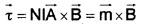3. θ = 0, τ = 0, Potential energy is minimum (Area vector of the coil is parallel to the direction of magnetic field.)

4. I = 4m
2πr = 4
r = $$\frac{4}{2 \pi}$$
Area = A = πr2
Maximum torque = NIAB = 1 × 1 × π × $$\left(\frac{4}{2 \pi}\right)^{2}$$ × 0.4
= 0.509 Nm

Question 4.
Ampere’s theorem helps to find the magnetic field in a region around a current carrying conductor.

1. Draw the variation of intensity of magnetic field with the distance from the axis of a current carrying conductor.
2. A conductor carrying a current I is bent as shown in the figure. Apply Ampere’s theorem at the regions 1 and 2 shown in the figure.1.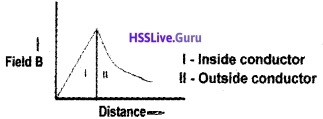2.Question 5.
The force acting on a moving charge in a magnetic field is called magnetic Lorents force.

1. Write the equation of force experienced by a charged particle moving in a magnetic field.
2. Using the equation derive an expression for the force acting on a current carrying conductor of length T in a magnetic field B.
3. “A charge can move in a helical path in a magnetic field”. Do you agree with it? Explain.

1.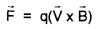2. Consider a rod of uniform cross section ‘A’ and length ‘ l ’. Let ’n’ be the number of electrons per unit volume (number density). ‘vd’ be the drift velocity of electrons for steady current ‘I’.
Total number of electrons in the entire volume of rod = nA l
Charge of total electrons = nA l .e
‘e’ is the charge of a single electron.
The Lorentz force on electrons,3. Yes, When an electron moves in a magnetic field with an angle θ, the electron undergoes for helical motion. The velocity of electron has two components, usinθ and ucosθ.

The component usinθ produces circular motion and ‘ucosθ’ produces translational motion. The combined effect of circular motion and translation motion will be helical motion.Question 6.
A long wire is bent into a circular coil of one turn having radius ‘R’ and a current T is passed through it

1. Name the law to find the direction of magnetic field due to this current loop
2. Find an expression for magnetic field produced by this current loop at its centre
3. If the same wire is bent to a smaller radius Y having ‘n’ turns and send same current through it. Find the ratio of magnetic field at the centre in two cases.

1. Right hand screw rule.

2. The magnetic field at a distance × from centre of loop is given by3.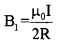If wire is bend into smaller radius of n turns.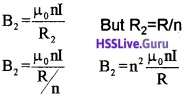B2 = n2 B1Question 7.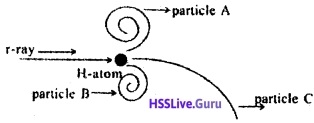A gas chamber is filled with hydrogen and a magnetic field is applied to it, then exposed to γ-ray. The γ-ray hits the hydrogen atom and produces high energy electron, low energy electron and positron (electron having + ve charge). The above photograph represents the trajectory of the particles. [Here magnetic field is applied Out of the plane of photo graph]

1. Which force drives the particle in a circular path and write the mathematical from.
2. Obtain a general expression for radius of the circular path.
3. Analyse the figure and match the columns given below.1. Magnetic Lorentz force

2. The centripetal force required for rotation is given magnetic Lorentz force Hence we can write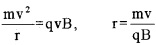3. Analyse the figure and match the columns given below:

• Low energy electrons – B
• High energy electron – C
• Positron – AQuestion 8.
A proton, an electron, a neutron, and an alpha particle are entering a region of uniform magnetic field with same velocities. The tracks of these particles are labelled.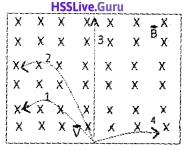1. Identify the tracks of each particle. (1)
2. Write the expression for the force experienced by a charged particle in the magnetic field in vector form. (1)
3. If the proton is moving at 90° to the uniform magnetic field what will be the change in kinetic energy of the proton? Give reason. (1)
4. An electron with energy 1 keV is entering a uniform magnetic field of 0.04T at an angle 60° with the field. Predict the path of the electron and find the characteristics of the path. (2)

1. Identify the tracks of each particle:

• Path 1 – proton
• Path 2 – alpha particle
• Path 3 – neutron
• Path 4 – electron

2.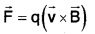3. Zero. Since the force is perpendicular to the direction of velocity work done is zero.

4. KE = 1 keV = 1 × 103 × 1.6 × 10-19 = 1.6 × 10-16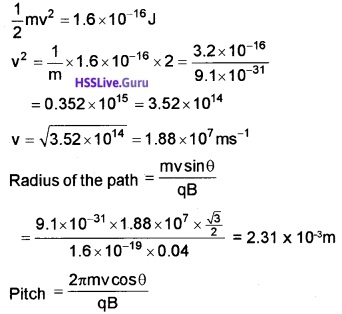= 8.39 × 10-3.Question 9.
A current carrying conductor is bent in the form of a circular ring and is placed in the plane of the paper.1. What is the direction of the magnetic field at the centre of the ring? (1)
2. Arrive at the expression for the magnetic field at a point on the axis of the ring. (3)
3. Another identical ring carrying the same current is brought with its axis perpendicular to the axis of the first as in figure. Find the magneticfield at the common centre. Calculate the angle between the net magnetic field and the axis of any one of the coils. (1)1. Out of the plane of the ring.

2.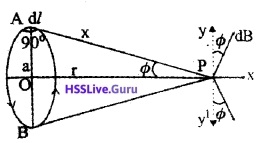Consider a circular loop of radius ‘a’ and carrying current ‘I’. Let P be a point on the axis of the coil, at distance x from A and r from ‘O’. Consider a small length dl at A. The magnetic field at ‘p’ due to this small element dl,[since sin 90° -1]
The dB can be resolved into dB cosΦ (along Py) and dB sinΦ (along Px). Similarly consider a small element at B, which produces a magnetic field ‘dB’ at P.If we resolve this magnetic field we get. dB sinΦ (along px) and dB cosΦ (along py1) dB cosΦ components cancel each other, because they are in opposite direction. So only dB sinΦ components are found at P, so total filed at P is
B = ∫dBsinΦbut from ∆AOP we get, sinΦ = a/x
∴ We get,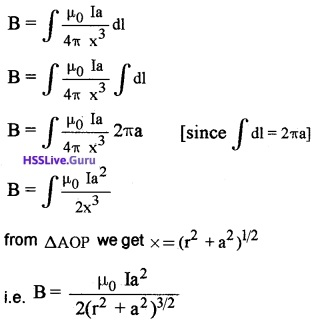Let there be N turns in the loop then,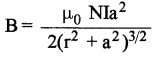At the center of the loop,
r= 0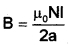3. Magnetic fields are as shown in the fig.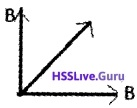The diagonal gives the resultant field.Question 10.
An electron revolving round the nucleus acts as a magnetic dipole.

1. Which force provides the centripetal force for electron? (1)
2. Write the expression for the magnetic dipole moment of electron in vector form. What is the angle between direction of the magnetic moment and direction of angular momentum? (2)
3. A charge 2mC is moving through a circular path of radius 0.15m with frequency 1000Hz. Find the magnetic moment associated with the charge path. (2)

1. Electrostatic force between electron and the nucleus.

2. VectoriallyThe magnetic moment of the electron is opposite in direction to the angular momentum.
∴ Angle = 180°.

3. Revolving charge behaves as a current loop. Hence Magnetic field is given asI = $$\frac{q}{T}$$ = qv = 2 × 10-3 × 100 = 0.2A
Magnetic moment = IA = 0.2 × π × 0.152
= 0.0141 Am.Question 11.
You are supplied with a galvanometer, resistor, and some connecting wires.

1. Using a circuit diagram, show how will you convert the given galvanometer into an ammeter. (1)
2. Find the expression for the shunt resistance in the circuit. (2)
3. A galvanometer is to be converted into an ammeter of range 0 -1 A. Galvanometer has resistance 100Ω and the current for full-scale deflection is 10mA. Find the length of the nichrome wire to be used as shunt. (3)

Given, Resistivity ρ = 1.1 × 10-6Ωm
Diameter of the wire = 1 mm